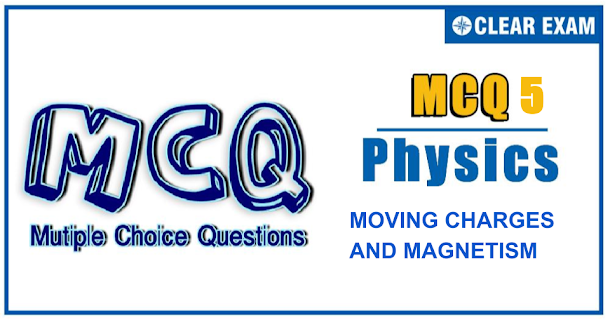## [LATEST]\$type=sticky\$show=home\$rm=0\$va=0\$count=4\$va=0

JEE Advanced Physics Syllabus can be referred by the IIT aspirants to get a detailed list of all topics that are important in cracking the entrance examination. JEE Advanced syllabus for Physics has been designed in such a way that it offers very practical and application-based learning to further make it easier for students to understand every concept or topic by correlating it with day-to-day experiences. In comparison to the other two subjects, the syllabus of JEE Advanced for physics is developed in such a way so as to test the deep understanding and application of concepts.

Q1.  A conducing ring of mass 2 kg and radius 0.5 m is placed on a smooth horizontal plane. The ring carries a current of i=4A. A horizontal magnetic field B=10 T is switched on at time t=0 as shown in Figure. The initial angular acceleration of the ring will be
Q2.A wire is bent in the form of a circular arc with a straight portion AB. Magnetic induction at O when current I flowing in the wire, is
•  μ_0/2r(π-θ+tan⁡θ)
•  (μ_0 I)/2πr(π+θ-tan⁡θ)
•  (μ_0 I)/2πr(π-θ+tan⁡θ)
•  (μ_0 I)/2πr(-tan⁡θ+π-θ)
Q3.  A consider a hypothetic spherical body. The body is cut into two parts about the diameter. One of hemispherical portion has mass distribution m whicle the other portion has identical charge distributionq. The body is rotated about the axis with constant speedω. Then, the ratio of magnetic moment to angular momentum is
•  q/2m
•  >q/2m
•  <q/2m
•  Cannot be calculated
Solution

Q4. A charged particle moves in a uniform magnetic field perpendicular to it, with a radius of curvature 4 cm. On passing through a metallic sheet it loses half of its kinetic energy. Then, the radius of curvature of the particle is
•  2cm
•  4cm
•  8cm
•  2√2 cm
Solution
Q5. A Q5.A charged particle is released from rest in a region of steady and uniform electric and magnetic fields which are parallel to each other. The particle will move in a

•  Straight line
•  circle
•  Helix
•  cycloid
Q6. Two concentric coils, each of radius equal to 2π cm, are placed at right angles to each other, Currents of 3A and 4A, respectively, are flowing through the two coils. The magnetic induction, in Wbm^(-2), at the centre of the coils will be

•  5×10^(-5)
•  7×10^(-5)
• 12×10^(-5)
•  10^(-5)
Q7.Same current i is flowing in three infinitely long wires along positive x-,y- and z-directions. The magnetic field at a point (0,0,-a) would be

•  (μ_0 i)/2πa(j ̂-i ̂)
•  (μ_0 i)/2πa(i ̂+j ̂)
•  (μ_0 i)/2πa(i ̂-j ̂)
•  (μ_0 i)/2πa(i ̂+j ̂+k ̂)
Q8.Two positive charge q_1 and q_2 are moving with velocities v_1 and v_2 when they are at points A andB, respectively, as shown in figure. The magnitude force experienced by charge q_1 due to the other charge q_2 is
•  (μ_0 q_1 q_2 v_1 v_2)/(8√2 πa^2 )
•  T(μ_0 q_1 q_2 v_1 v_2)/(4√2 πa^2 )
•  (μ_0 q_1 q_2 v_1 v_2)/(2√2 πa^2 )
•  (μ_0 q_1 q_2 v_1 v_2)/(√2 πa^2 )
Q9.An otherwise infinite, straight wire has two concentric loops os radii a and b carrying equal currents in opposite directions as shown in figure. The magnetic field at the common centre is zero for
•  a/b=(π-1)/π
•  a/b=π/(π+1)
•  a/b=(π-1)/(π+1)
•  a/b=(π+1)/(π-1)
Q10. The magnitude field at O due to current in the infinite wire forming a loop as shown in figure is
•  (μ_0 I)/4πd(cos⁡〖ϕ_1+cos⁡〖ϕ_2 〗 〗)
•  μ_0/4π 2I/d(tan⁡〖θ_1 〗+〖tan⁡θ〗_2)
•  μ_0/4π I/d(sin⁡〖ϕ_1+sin⁡〖ϕ_2 〗 〗)
• μ_0/4π 2I/d(cos⁡〖θ_1 〗+〖cos⁡θ〗_2)
Solution#### Written by: AUTHORNAME

AUTHORDESCRIPTION## Want to know more

Please fill in the details below:

## Latest NEET Articles\$type=three\$c=3\$author=hide\$comment=hide\$rm=hide\$date=hide\$snippet=hide

Name

ltr
item
BEST NEET COACHING CENTER | BEST IIT JEE COACHING INSTITUTE | BEST NEET & IIT JEE COACHING: Moving Charges and Magnetism-Quiz-5
Moving Charges and Magnetism-Quiz-5
https://1.bp.blogspot.com/-YxeWSKZlepc/YOcp9bBvJVI/AAAAAAAAAK4/py55zUNkjZ8lMV4o1gbjGjX3U-3oCM60QCLcBGAsYHQ/w612-h320/Quiz%2BImage%2B20.png
https://1.bp.blogspot.com/-YxeWSKZlepc/YOcp9bBvJVI/AAAAAAAAAK4/py55zUNkjZ8lMV4o1gbjGjX3U-3oCM60QCLcBGAsYHQ/s72-w612-c-h320/Quiz%2BImage%2B20.png
BEST NEET COACHING CENTER | BEST IIT JEE COACHING INSTITUTE | BEST NEET & IIT JEE COACHING
https://www.cleariitmedical.com/2021/07/moving-charges-and-magnetism-quiz-5.html
https://www.cleariitmedical.com/
https://www.cleariitmedical.com/
https://www.cleariitmedical.com/2021/07/moving-charges-and-magnetism-quiz-5.html
true
7783647550433378923
UTF-8

STAY CONNECTED